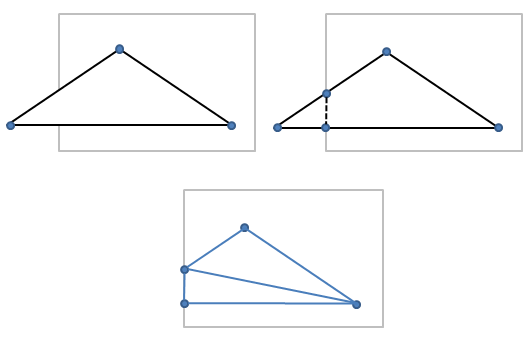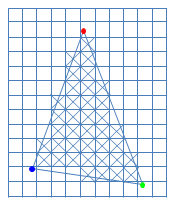Prev: GS Examples Next: Fragment Shader

This section deals with two important concepts for understading graphic pipelines: rasterization and interpolation. These are fixed components of the graphics pipeline, i.e. they are not programmable.

However before rasterization occurs, there are a few small steps that will be described now, namely clipping transformation to window coordinates.

Clipping

This stage receives the assembled primtives, with transformed vertices, and checks if they are inside the clip volume. Primitives totally inside the clipping volume are not altered. Primitives outside the viewing volume are discarded. Primitives whose edges intersect the boundaries of the clipping volume are clipped.

The clip volume is a transformation of the view frustum such that all vertices that are inside the view frustum have coordinates$(x_c,y_c,z_c,w_c)$ such that$-w_c \leq x_c \leq w_c$$-w_c \leq y_c \leq w_c$$-w_c \leq z_c \leq w_c$

The gl_Position atribute of the vertex holds the clip coordinates and is tested for clipping purposes. The vertex clip coordinates are$(x_c,y_c,z_c,w_c)$.

For primtives that have edges where the vertices are on opposite sides of a clipping volume delimiting plane, a new vertex is computed on the intersection point for each clipping plane that is intersected. These new vertices will become part of the primitive, and the vertices outside the clipping volume are discarded. Therefore, clipping may introduce new vertices on a triangle primitive, but that is transparent to the programmer.

The following figure shows what happens to a triangle partly outside the clip volume.The new vertices have the associated attributes interpolated along the respective edge, unless flat shading is specified in the attributes declaration in which case the value of the attribute is constant along the edge.

The vertex can then be transformed, a.k.a. perspective division, to normalized device coordinates, i.e.$(x_d, y_d, z_d) = (x_c/w_c, y_c/w_c, z_c/w_c)$

Considering normalized device coordinates the clip volume becomes a 3D cube that ranges from -1 to 1 in all its coordinates (unless depth clamping is disabled in which case there is no limitation for the z values). Hence, a vertex will be inside the clipping volume, with depth clamping, if:$-1 \leq x_d \leq 1$$-1 \leq y_d \leq 1$$-1 \leq z_d \leq 1$

When specifying flat in a declaration of a vertex attribute, the attribute will be copied form one of the vertices to all the remaining vertices of the primitive. If nothing is said, then the last vertex of the primitive will determine the value of the attribute for all the other vertices. This vertex is called the provoking vertex. OpenGL allows us to specify the first or last vertex as the provoking vertex using the command:

glProvokingVertex(enum provokeMode);

where provokeMode can have the following values: GL_FIRST_VERTEX_CONVENTION or GL_LAST_VERTEX_CONVENTION (this last value is the default).

Window Coordinates

Window coordinates can now be computed for every vertex. The window coordinates are dependent on the viewport. Consider a viewport with height h, width w and center in$(o_x, o_y)$. All these values are in window coordinates and the units are pixels.

A vertex with normalized device coordinates$(x_d,y_d,z_d)$ will have window coordinates$(x_w, y_w, z_w)$ computed as:$(x_w, y_w, z_w) = (x_d*(w/2) + o_x, y_d * ( h/2) + o_y, (z_d * (f-n) + (n+f))/2)$

where n and f stand for the near and far values that may be specified with the glDepthRange* family of OpenGL functions. In not specified, then n = 0 and f = 1, and the conversion above simplifies to:$(x_w, y_w, z_w) = (x_d*(w/2) + o_x, y_d * ( h/2) + o_y, (z_d + 1)/2)$

Rasterization

Rasterization is the process of determining the set of pixels, in the final image, that are part of the primitive.

The first step of polygon rasterization is to determine its orientation, i.e. is it front or back facing. If culling is enable, all those triangles that have not the proper orientation are discarded, and the rasterization process ends for them.

For correctly oriented triangles, we have to determine the pixels that are part of it. Each pixel whose center is inside the triangle boundaries will be added to the set of pixels for further processing. In the figure below, the colored dots represent the vertices position in screen space. Note that the pixel where the vertex lies may not be part of the set of pixels that make up the triangle.Interpolation

The next step is computing the attributes for each pixel based on the vertex attributes and the pixel’s distance to each vertex screen position.

Barycentric coordinates are used to help with the interpolation process. Any point p inside a triangle (pa,pb,pc) can be expressed as:$p = a * pa + b * pb + c * pc$

where$a + b + c = 1$

and$0 \leq a \leq 1$$0 \leq b \leq 1$$0 \leq c \leq 1$

The triplet (a, b, c) contains the barycentric coordinates of point p. The barycentric coordinates can be seen as weights that determine the influence that each vertex has on a point inside the triangle, and, as mentioned before, the sum of the weights is equal to 1.

For each pixel, the value of each attribute is interpolated considering the point p with coordinates that represent the center of the pixel and taking into account the weights computed previously, i.e. the barycentric coordinates, and the values of the attributes at the vertices in screen space.Prev: GS Examples Next: Fragment Shader

### 2 Responses to “GLSL Tutorial – Rasterization and Interpolation”

1.I believed it should be (f – n) / 2 in “Window Coordinates”.

•I’m not sure I’m getting your point. The formula divides everything by 2 and it is as presented in the OpenGL spec. Can you please be more specific?

This site uses Akismet to reduce spam. Learn how your comment data is processed.